# tensorflow学习笔记——自编码器及多层感知器

### 1，自编码器简介

传统机器学习任务很大程度上依赖于好的特征工程，比如对数值型，日期时间型，种类型等特征的提取。特征工程往往是非常耗时耗力的，在图像，语音和视频中提取到有效的特征就更难了，工程师必须在这些领域有非常深入的理解，并且使用专业算法提取这些数据的特征。深度学习则可以解决人工难以提取有效特征的问题，它可以大大缓解机器学习模型对特征工程的依赖。深度学习在早期一度被认为是一种无监督的特征学习（Unsuperbised Feature Learning），模仿了人脑的对特征逐层抽象提取的过程。这其中有两点很重要：一是无监督学习，即我们不需要标注数据就可以对数据进行一定程度的学习，这种学习是对数据内容的组织形式的学习，提取的时频繁出现的特征；二是逐层抽象，特征是需要不断抽象的，就像人总是从简单基础的概念开始学习，再到复杂的概念。学生们要从加减乘除开始学起，再到简单函数，然后到微积分，深度学习也是一样的，它从简单的微观的特征开始，不断抽象特征的层级，逐渐往复杂的宏观特征转变。

例如在图像识别问题中，假定我们有许多汽车的图片，要如何判定这些图片是汽车呢？如果我们从像素级特征开始进行训练分类器，那么绝大多数算法很难有效的工作。如果我们提取出高阶的特征，比如汽车的车轮，汽车的车窗，汽车的车身，那么使用这些高阶特征便可以非常准确地对图片进行分类，这就是高阶特征的效果。不过任何高阶特征都是由更小单位的特征组合而成的。比如车轮是由橡胶轮胎，车轴，轮辐等组成。而其中每一个组件都是由更小单位的特征组合而成的，比如橡胶轮胎由许多黑色的同心圆组成的，而这些同心圆也都由许多圆弧曲线组成，圆弧曲线都由像素组成。我们将漆面的过程逆过来，将一张图片的原始像素慢慢抽象，从像素组成点，线，再讲点，线组合成小零件，再将小零件组成车轮，车窗，车身等高阶特征，这边是深度学习在训练过程中所作的特征学习。

早年由学者们研究稀疏编码（Sparse Coding）时，他们收集了大量黑白风景照，并从中提取了许多16像素*16像素的图像碎片。他们发现几乎所有的图像碎片都可以由64种正交的边组合得到，如下图所示，并且组合出一张图像碎片需要的边的数量是很少的，即稀疏的。学者同时发现声音也存在这种情况，他们从大量的未标注音频中发现了20种基本结构，绝大多数声音可以由这些基本结构线性组合得到。这其实就是特征的稀疏表达，使用少量的基本特征组合拼装得到更高层抽象的特征，通常我们也需要多层的神经网络，对每一层神经网络来说，前一层的输出都是未加工的像素，而这一层则是对象素进行加工组织成更高阶的特征（即前面提到的将边组合成图像碎片）。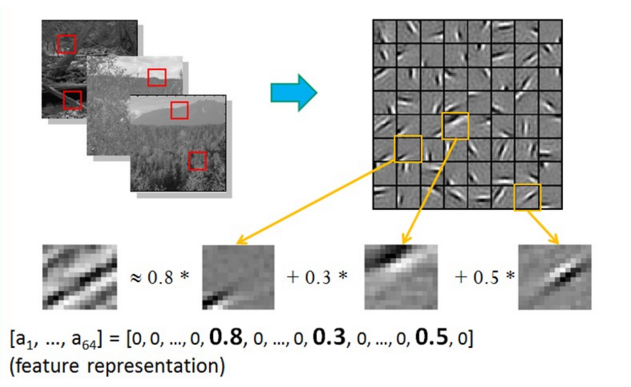我们来看一下实际的例子。假如我们有许多基本结构，比如指向各个方向的边，白块，黑块等。如下图所示，我们可以通过不同方式组合出不同的高阶特征，并最终拼出不同的目标物体。这些基本结构就是basis，在人脸识别任务中，我们可以使用他们拼出人脸的不同器官，比如鼻子，嘴，眼睛，眉毛，脸颊等，这些器官又可以向上一层拼出不同样式的人脸，最后模型通过在图片中匹配这些不同样式 的人脸（即高阶特征）来进行识别。同样，basis可以拼出汽车上不同的组件，最终拼出各式各样的车型；也可以拼出大象身体的不同部位，最后组成各种尺寸，品种，颜色的大象；还可以拼出椅子的凳，座，靠背凳，最后组成各种尺寸，品种，颜色的大象；还可以拼出椅子的凳，座，靠背凳，最后组成不同款式的椅子，特征是可以不断抽象转为高一级的特征的，那我们如何找到这些基本结构，然后如何抽象呢？如果我们有很多标注的数据，则可以训练一个深层的神经网络。如果没有标注的数据呢？在这种情况下，我们依然可以使用无监督的自编码器来提取特征。自编码器（AutoEncoder），顾名思义，即可以使用自身的高阶特征编码自己。自编码器其实也是一种神经网络，它的输入和输出是一致的，它借助稀疏编码的思想，目标是使用稀疏的一些高阶特征重新组合来重构自己。因此，它的特点非常明显：第一，期望输入/输出一致；第二，希望使用高阶特征来重构自己，而不是复制像素点。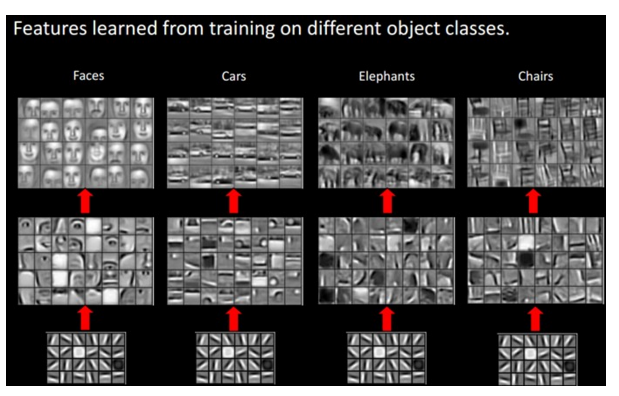Hinton 教授在Science发表文章 Reducing the dimensionality of data with neural networks，讲解了使用自编码器对数据进行降维的方法。Hinton还提出了基于深度信念网络（Deep Belief Networks， DBN，由多层RBM堆叠而成）可使用无监督的逐层训练的贪心算法，为训练很深的网络提供一个可行方案：我们可能很难直接训练极深的网络，但是可以用无监督的逐层训练提取特征，将网络的权重初始化到一个比较好的位置，辅助后面的监督训练。无监督的逐层训练，其思想和自编码器（AutoEncoder）非常相似，后者的目标是让神经网络的输出能和原始输入一致，相当于学习一个恒等式 y=x， 如下图所示，自编码器的输入节点和输出节点的数量是一致的，但如果只是单纯的逐个复制输入节点则没有意义，像前面提到的，自编码器通常希望使用少量稀疏的高阶特征来重构输入，所以我们可以加入几种限制。

（1）如果限制中间隐含层节点的数量，比如让中间隐含层节点的数量小于输入/输出节点的数量，就相当于一个降维的过程。此时已经不可能出现复制所有节点的情况，因为中间节点数小于输入节点数，那只能学习数据中最重要的特征复原，将可能不太相关的内容去掉。此时，如果再给中间隐含层的权重加一个L1的正则，则可以根据惩罚系数控制隐含节点的稀疏程度，惩罚系数越大，学到的特征组合越稀疏，实际使用（非零权重）的特征数量越少。

（2）如果给数据加入噪音，那么就是Denoising AutoEncoder（去噪自编码器），我们将从噪声中学习出数据的特征。同样，我们也不可能完全复制节点，完全复制并不能去除我们添加的噪声，无法完全复原数据，所以唯有学习数据频繁出现的模式和结构，将无规律的噪声略去，才可以复原数据。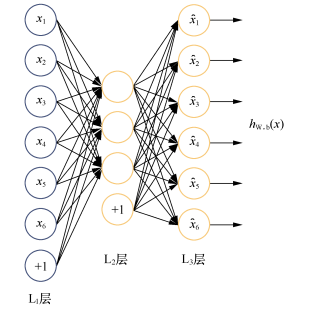### 2，TensorFlow实现自编码器

这里我们主要来自TensorFlow的开源实现，使用的数据为MNIST数据。我们的自编码器会使用到一种参数初始化方法 xavier initialization，需要先定义好它。Xavier初始化器在Caffe的早期版本中被频繁使用，它的特点是会根据某一层网络的输入，输出节点数量自动调整最适合的分布。Xaiver Glorot 和深度学习三巨头之一的 Yoshua Bengio 在一篇论文中指出，如果深度学习模型的权重初始化的太小，那信号将在每层间传递时逐渐缩小而难以产生作用，但如果权重初始化的太大，那信号将在每层间传递时逐渐放大并导致发散和实效。而 Xavier 初始化器做的事情就是让权重被初始化的不大不小，正好合适。从数学的角度分析， Xavier 初始化器做的事情就是让权重被初始化的不大不小，正好合适。从数学的角度分析， Xavier 初始化器就是让权重满足0均值，同时方差为 2/(Nin + Nout)，分布可以用均匀分布或者高斯分布。如下代码：

def xavier_init(fan_in, fan_out, constant = 1):
low = -constant * np.sqrt(6.0 / (fan_in + fan_out))
high = constant * np.sqrt(6.0 / (fan_in + fan_out))
return tf.random_uniform((fan_in, fan_out),
minval=low, maxval=high,
dtype=tf.float32)


其中 fan_in 是输入节点的数量，fan_out 是输出节点的数量。我们使用了 tf.random_uniform创建了一个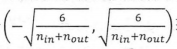范围内的均匀分布，而它的方差根据公式 D(x) = (max - min) **2 /12刚好等于 2/(Nin + Nout)。因此这时实现的就是标准的均匀分布的 Xaiver 初始化器。

代码如下：

#_*_coding:utf-8_*_
import numpy as np
import sklearn.preprocessing as prep
import tensorflow as tf
from tensorflow.examples.tutorials.mnist import input_data

def xavier_init(fan_in, fan_out, constant = 1):
low = -constant * np.sqrt(6.0 / (fan_in + fan_out))
high = constant * np.sqrt(6.0 / (fan_in + fan_out))
return tf.random_uniform((fan_in, fan_out),
minval=low, maxval=high,
dtype=tf.float32)

def __init__(self, n_input, n_hidden, transfer_function=tf.nn.softplus,
self.n_input = n_input           # 输入变量数
self.n_hidden = n_hidden         # 隐含层节点数
self.transfer = transfer_function  # 隐含层激活函数
self.scale = tf.placeholder(tf.float32)  #高斯噪声稀疏
self.training_scale = scale
network_weights = self._initialize_weights()   # 我们只用了一个隐含层
self.weights = network_weights

self.x = tf.placeholder(tf.float32, [None, self.n_input])
self.x + scale * tf.random_normal((n_input, )),
self.weights['w1']), self.weights['b1']
))
self.weights['w2']),
self.weights['b2'])
self.cost = 0.5 * tf.reduce_sum(tf.pow(tf.subtract(
self.reconstruction, self.x), 2.0
))
self.optimizer = optimizer.minimize(self.cost)

init = tf.global_variables_initializer()
self.sess = tf.Session()
self.sess.run(init)

def _initialize_weights(self):
all_weights = dict()
all_weights['w1'] = tf.Variable(xavier_init(self.n_input, self.n_hidden))
all_weights['b1'] = tf.Variable(tf.zeros([self.n_hidden], dtype=tf.float32))
all_weights['w2'] = tf.Variable(tf.zeros([self.n_hidden,
self.n_input], dtype=tf.float32))
all_weights['b2'] = tf.Variable(tf.zeros([self.n_input], dtype=tf.float32))
return all_weights

# 计算损失cost及执行一步训练的函数partial_fit
def partial_fit(self, X):
cost, opt = self.sess.run((self.cost, self.optimizer),
feed_dict={self.x: X, self.scale: self.training_scale})
return cost

# 让session执行一个计算图节点self.cost，在测试会用到
def calc_total_cost(self, X):
return self.sess.run(self.cost,
feed_dict={self.x: X, self.scale: self.training_scale})

# 返回自编码器隐含层的输出结果，目的是提供一个接口来获取抽象后的特征
def transform(self, X):
return self.sess.run(self.hidden, feed_dict={self.x: X,
self.scale: self.training_scale})

# generate函数，将隐含层的输出结果作为输入
# 通过之后的重建层将提取到的高阶特征复原为原始数据
def generate(self, hidden = None):
if hidden is None:
hidden = np.random.normal(size=self.weights['b1'])
return self.sess.run(self.reconstruction, feed_dict={self.hidden:hidden})

# 整体运行一遍复原过程，包括提取高阶特征和通过高阶特征复原数据
def reconstruct(self, X):
return self.sess.run(self.reconstruction, feed_dict={self.x: X,
self.scale: self.training_scale})

# 获取隐含层的权重 W1
def getWeights(self):
return self.sess.run(self.weights['w1'])

# 获取隐含层的偏置系数 b1
def getBiases(self):
return self.sess.run(self.weights['b1'])

def standard_scale(X_train, X_test):
# 标准化即让数据变成0均值，且标准差为1的分布
preprocessor = prep.StandardScaler().fit(X_train)
X_train = preprocessor.transform(X_train)
X_test = preprocessor.transform(X_test)
return X_train, X_test

# 定义一个获取随机block数据的函数，取一个从0到len(data)-batch_size之间的随机整数
# 这里是不放回抽样，可以提高数据的利益效率
def get_random_block_from_data(data, batch_size):
start_index = np.random.randint(0, len(data) - batch_size)
return data[start_index: (start_index + batch_size)]

# 使用之前定义的standard_scale 函数对训练集，测试集进行标准化变换
X_train, X_test = standard_scale(mnist.train.images, mnist.test.images)

n_samples = int(mnist.train.num_examples)  # 总训练样本数据
training_epochs = 20   # 最大训练的轮数
batch_size = 128   # 批次尺寸
display_step = 1

n_hidden=200,
transfer_function=tf.nn.softplus,
scale=0.01)

for epoch in range(training_epochs):
avg_cost = 0
total_batch = int(n_samples / batch_size)
for i in range(total_batch):
batch_xs = get_random_block_from_data(X_train, batch_size)

cost = autoencoder.partial_fit(batch_xs)
avg_cost += cost / n_samples * batch_size

if epoch % display_step == 0:
print("Epoch:", '%04d' %(epoch + 1), 'cost=', "{:.9f}".format(avg_cost))

print("Total cost: " + str(autoencoder.calc_total_cost(X_test)))


结果如下：

Epoch: 0001 cost= 19994.269430682
Epoch: 0002 cost= 12152.192460227
Epoch: 0003 cost= 10875.503042045
Epoch: 0004 cost= 10308.418541477
Epoch: 0005 cost= 9187.305378977
Epoch: 0006 cost= 9217.541369886
Epoch: 0007 cost= 9685.120126136
Epoch: 0008 cost= 9682.280556250
Epoch: 0009 cost= 8886.662347727
Epoch: 0010 cost= 8706.033726705
Epoch: 0011 cost= 7810.424784091
Epoch: 0012 cost= 8669.721145455
Epoch: 0013 cost= 8534.551150568
Epoch: 0014 cost= 7741.216421591
Epoch: 0015 cost= 7924.397942045
Epoch: 0016 cost= 7475.140959091
Epoch: 0017 cost= 8064.848693750
Epoch: 0018 cost= 8351.518777273
Epoch: 0019 cost= 7844.633439773
Epoch: 0020 cost= 8505.549214773
Total cost: 634369.4


上面为训练结果，我们将平均损失 avg_cost 设为0，并计算总共需要的 batch 数（通常样本总数除以batch大小），注意这里使用的时不放回抽样，所以并不能保证每个样本都被抽样并参与训练。然后在每一个 batch 的循环中，先使用  get_random_block_from_data 函数随机抽取一个 block 的数据，然后使用成员函数 partial_fit 训练这个 batch 的数据并计算当前的 cost，最后将当前的 cost 整合到 avg_cost 中，在每一轮迭代后，显示当前的迭代数和这一轮迭代的平均 cost。我们在第一轮迭代时，cost 大约为19999，在最后一轮迭代时， cost大约为7000，再接着训练 cost 也很难继续降低了。当然可以继续调整 batch_size，epoch数，优化器，自编码器的隐含层数，隐含节点数等，来尝试获取更低的 cost 。

最后对训练完的模型进行性能测试，这里使用之前定义的成员函数 cal_total_cost 对测试集 X_test 进行测试，评价指标依然是平方误差，如果使用示例中的参数，我们可以看到损失值为 60万。

至此，去噪自编码器的 TensorFlow完全实现。我们可以发现实现自编码器和实现一个单隐含层的神经网络差不多，只不过是在数据输入时做了标准化，并加上了一个高斯噪声，同时我们在输出结果不是数字分类结果，而是复原的数据，因此不需要用标注过的数据进行监督训练。自编码器作为一种无监督学习的方法，它与其他无监督学习的主要不同在于，它不是对数据进行聚类，而是提取其中最有用，最频繁出现的高阶特征，根据这些高阶特征重构数据。在深度学习发展早期非常流行的 DBN，也是依靠这种思想，先对数据进行无监督的学习，提取到一些有用的特征，将神经网络权重初始化到一个较好的分布，然后再使用有标注的数据进行监督训练，即对权重进行 fine-tune。

现在，无监督式预训练的使用场景比以前少了许多，训练全连接的 MLP 或者 CNN，RNN 时，我们都不需要先使用无监督训练提取特征。但是无监督学习乃至 AutoEncoder 依然是非常有用的。现实生活中，大部分的数据都是没有标注信息的，但人脑就很擅长处理这些数据，我们会提取其中的高阶抽象特征，并使用在其他地方。自编码器作为深度学习在无监督领域的尝试是非常成功的，同时无监督学习也将是深度学习接下来的一个重要发展方向。

### 3，pytorch实现自动编码器

#### 3.1  什么是自动编码器

自动编码器（AutoEncoder）最开始作为一种数据的压缩方法，其特点有：

（1） 跟数据相关程序很高，这意味着自动编码器只能压缩与训练数据相似的数据，这个其实比较显然，因为使用神经网络提取的特征一般是高度相关于原始的训练集，使用人脸训练出来的自动编码器在压缩自然界动物的图片是表现就会比较差，因为它只学习到了人脸的特征，而没有能够学习到自然界图片的特征；

（2）压缩后的数据是有损的，这是因为在降维过程中不可避免的要丢掉信息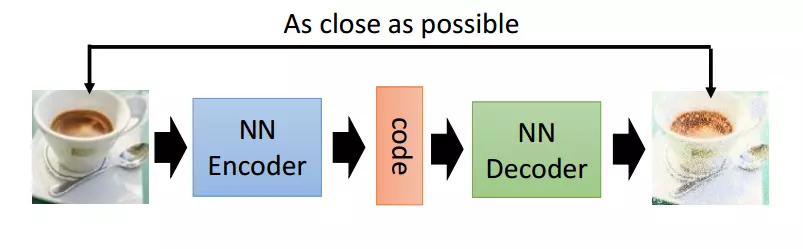Autoencoder 简单来说就是将有很多Feature的数据进行压缩，之后再进行解压的过程。比如有一个神经网络，它在做的事情是接收一张图片，然后给他打码，最后，再从打码后的图片中还原，如下图：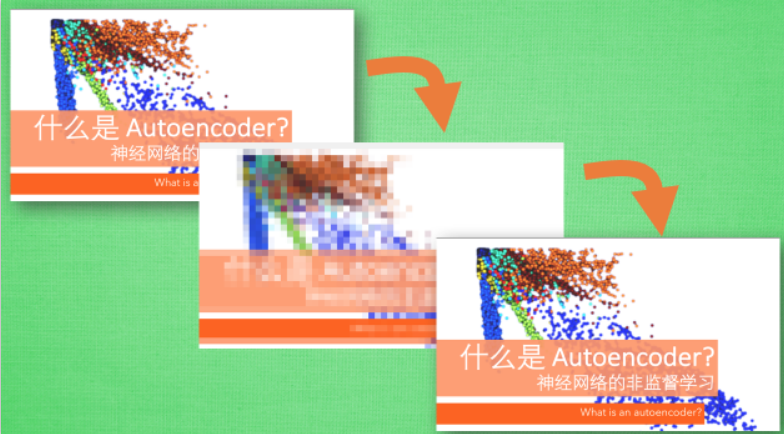因为有时候神经网络要接受大量的输入信息，比如输入信息是高清图片时，输入信息量可能达到上千万，让神经网络直接从上千万个信息源中学习是一件很吃力的工作。所以，压缩一下，提取出原图片中的最具有代表性的信息，缩减输入信息量，再将缩减过后的信息放入神经网络学习，这样学习就简单轻松了。

对于此问题来说，自编码在这时候就能发挥作用，通过将原数据白色的X压缩，解压成黑色的X，然后通过对比黑白X，求出预测误差，进行反向传递，逐步提升自编码的准确性。训练好的自编码中间这一部分就是能总结元数据的精髓。

对于自编码，我们只用了输入数据X，并没用到X对应的数据标签，所以也可以说自编码是一种非监督学习。如果大家知道PCA（Principal component analysis），与Autoencoder相类似，它的主要功能即使对数据进行非监督学习，并将压缩站会后得到的“特征值”，这一中间结果正类似于PCA的结果。之后再将压缩过的“特征值”进行解压，得到的最终结果与原始数据进行比较，对此进行非监督学习。其大概过程如下：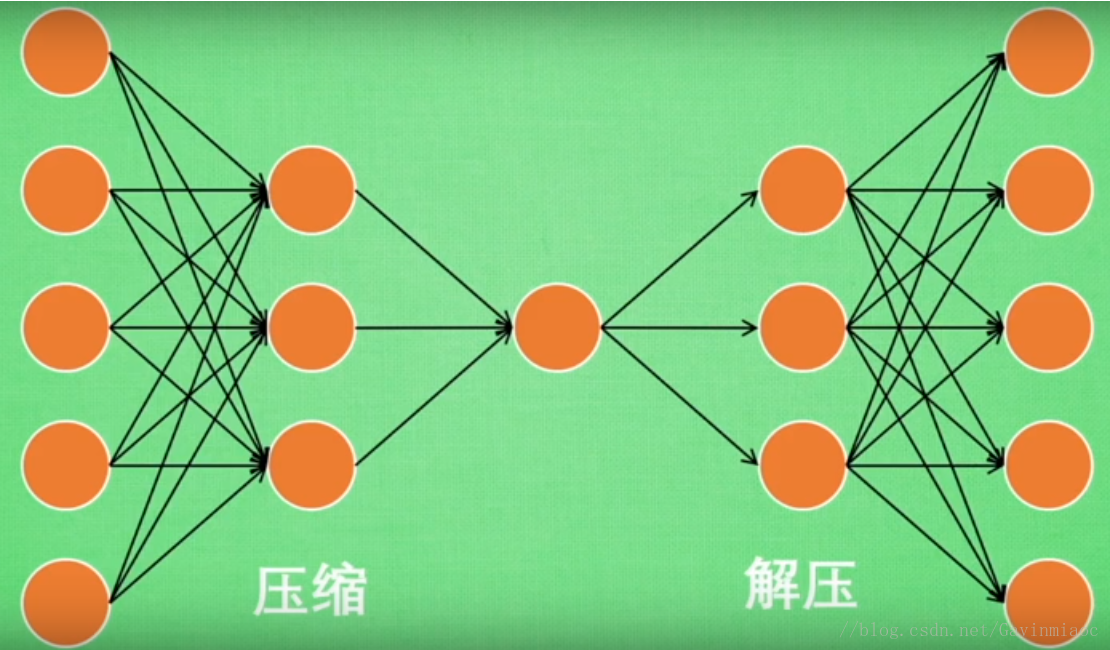这是一个通过自编码整理出来的数据，它能从元数据中总结出每种类型数据的特征，如果把这些特征类型都放在一张二维的图片上 ，每种类型都已经很好地用原数据的精髓区分来。如果你了解PCA主成分，再提取主要特征时，自编码和他一样，甚至超越了PCA，换句话说，自编码可以像PCA一样给特征属性降维。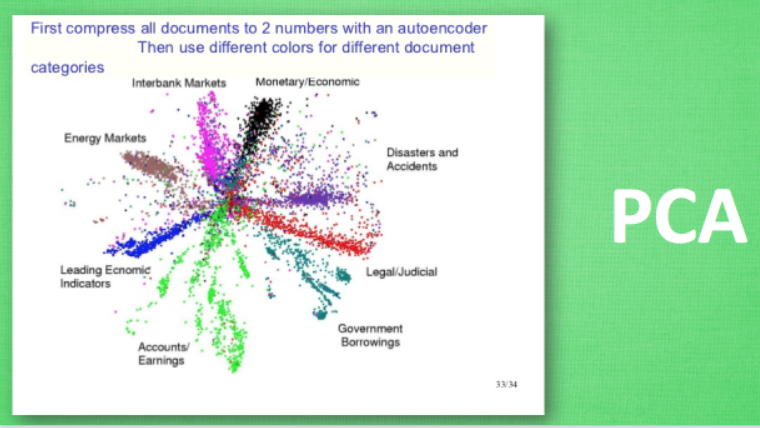#### 3.2  编码器的学习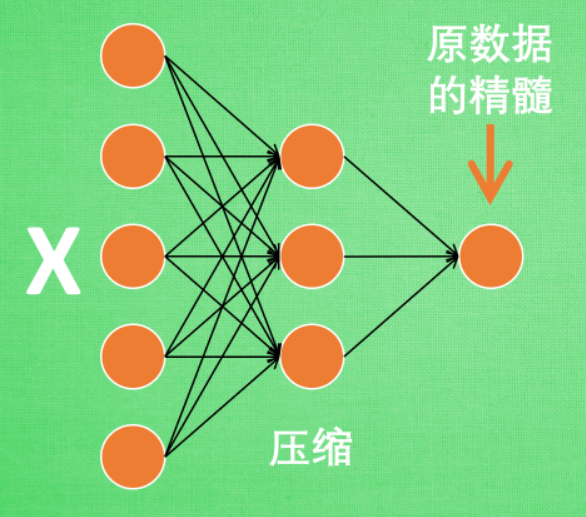这部分叫做encoder编码器，编码器能得到元数据的精髓，然后我们只需要再创建一个小的神经网络学习这个精髓的数据，不仅减少了神经网络的负担，同样也能达到很好的效果。

#### 3.3  解码器的学习

至于解码器Decoder，我们知道，它在训练的时候是要将精髓信息解压成原始信息，那么这就提供了一个解压器的作用，甚至我们可以认为是一个生成器（类似于GAN），那做这件事的一种特殊自编码叫做variational  autoencoders。

有一个例子就是让它能模仿并生成手写数字：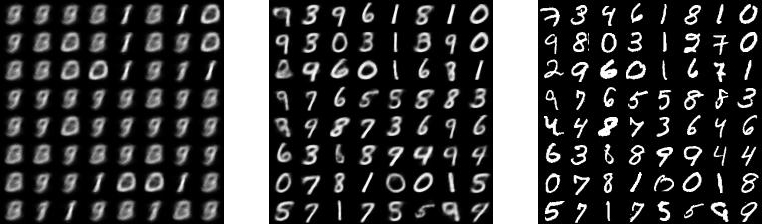#### 3.4  代码解析

今天的代码，我们会运用两个类型：

• 1，是通过Feature的压缩并解压，并将结果与原始数据进行对比，观察处理过后的数据是不是如预期跟原始数据很相似（这里会用到MNIST数据）
• 2，我们只看encoder压缩的过程，使用它将一个数据集压缩到只有两个Feature时，将数据放入一个二维坐标系内。

神经网络也能进行非监督学习，只需要训练数据，不需要标签数据。自编码就是这样一种形式。自编码能自动分类数据，而且也能嵌套在半监督学习的上面，用少量的有标签样本和大量的无标签样本学习。

这次学习用MNIST手写数据来压缩再解压图片。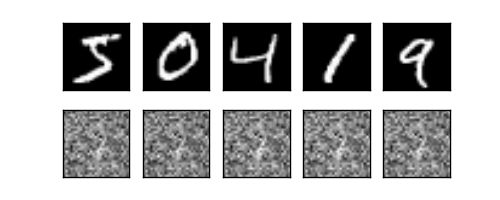然后用压缩的特征进行非（无）监督分类。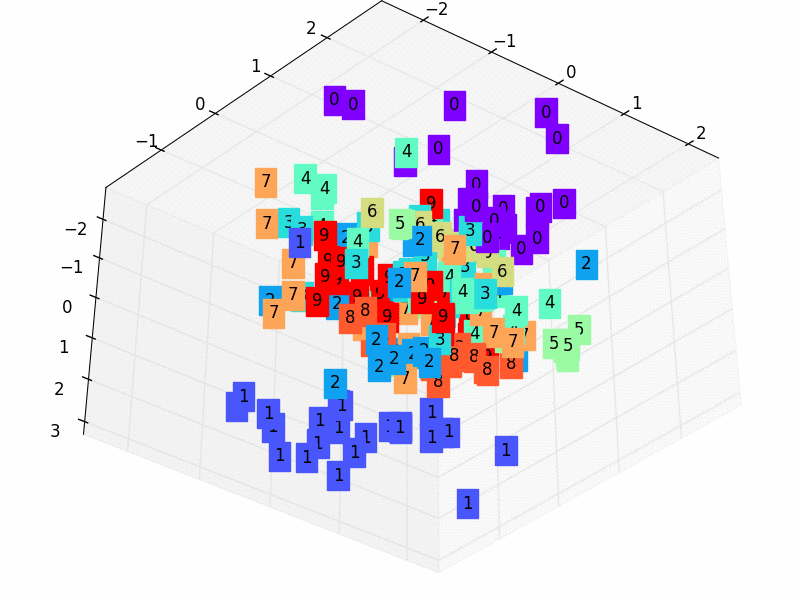#### 3.5  训练数据

自编码只能用训练集就好了，而且只需要训练training  data 的 image， 不用训练labels。

import torch
import torch.nn as nn
import torch.utils.data as Data
import torchvision

# 超参数
EPOCH = 10
BATCH_SIZE = 64
LR = 0.005
# 下过数据的话, 就可以设置成 False
# 到时候显示 5张图片看效果, 如上图一
N_TEST_IMG = 5

# Mnist digits dataset
train_data = torchvision.datasets.MNIST(
root='./mnist/',
# this is training data
train=True,
# Converts a PIL.Image or numpy.ndarray to
# torch.FloatTensor of shape (C x H x W) and normalize in the range [0.0, 1.0]
transform=torchvision.transforms.ToTensor(),
)


#### 3.6  AutoEncoder

AutoEncoder 的形式很监督，分别是encoder 和 decoder，压缩和解压，压缩后得到压缩的特征值，再从压缩的特征值解压成原图片。

class AutoEncoder(nn.Module):
def __init__(self):
super(AutoEncoder, self).__init__()

# 压缩
self.encoder = nn.Sequential(
nn.Linear(28*28, 128),
nn.Tanh(),
nn.Linear(128, 64),
nn.Tanh(),
nn.Linear(64, 12),
nn.Tanh(),
nn.Linear(12, 3),   # 压缩成3个特征, 进行 3D 图像可视化
)
# 解压
self.decoder = nn.Sequential(
nn.Linear(3, 12),
nn.Tanh(),
nn.Linear(12, 64),
nn.Tanh(),
nn.Linear(64, 128),
nn.Tanh(),
nn.Linear(128, 28*28),
nn.Sigmoid(),       # 激励函数让输出值在 (0, 1)
)

def forward(self, x):
encoded = self.encoder(x)
decoded = self.decoder(encoded)
return encoded, decoded

autoencoder = AutoEncoder()


这里我们定义了一个简单的四层网络作为编码器，中间使用ReLU激活函数，最后输出的是三维的。输出一个28 * 28 的图像数据，特别要注意的是最后使用的激活函数是Tanh，这个激活函数能够将最后的输出转换到 -1 和 1 之间，这是因为我们输入的图片已经变换到 -1 和 1 之间了，这是的输出必须和其对应。

我们也可以将多层感知器换成卷积神经网络，这样对图片的特征提取有着更好的效果。

class autoencoder(nn.Module):
def __init__(self):
super(autoencoder, self).__init__()
self.encoder = nn.Sequential(
nn.Conv2d(1, 16, 3, stride=3, padding=1),  # b, 16, 10, 10
nn.ReLU(True),
nn.MaxPool2d(2, stride=2),  # b, 16, 5, 5
nn.Conv2d(16, 8, 3, stride=2, padding=1),  # b, 8, 3, 3
nn.ReLU(True),
nn.MaxPool2d(2, stride=1)  # b, 8, 2, 2
)
self.decoder = nn.Sequential(
nn.ConvTranspose2d(8, 16, 3, stride=2),  # b, 16, 5, 5
nn.ReLU(True),
nn.ConvTranspose2d(16, 8, 5, stride=3, padding=1),  # b, 8, 15, 15
nn.ReLU(True),
nn.ConvTranspose2d(8, 1, 2, stride=2, padding=1),  # b, 1, 28, 28
nn.Tanh()
)

def forward(self, x):
x = self.encoder(x)
x = self.decoder(x)
return x


这里使用了 nn.ConvTranspose2d()，这可以看做是卷积的反操作，可以在某种意义上看做是反卷积。

#### 3.7  训练并可视化

训练，并可视化训练的过程，我们可以有效的利用encoder 和decoder来做很多事情，比如这里我们用decoder的信息输出看和原图片的对比，还能用encoder来看经过压缩后，神经网络对原图片的理解。encoder能将不同图片数据大概的分离开来，这样就是一个无监督学习的过程。而训练过程也比较简单，我们使用最小均方误差来作为损失函数，比较生成的图片与原始图片的每个像素点的差异。

optimizer = torch.optim.Adam(autoencoder.parameters(), lr=LR)
loss_func = nn.MSELoss()

for epoch in range(EPOCH):
for step, (x, b_label) in enumerate(train_loader):
b_x = x.view(-1, 28*28)   # batch x, shape (batch, 28*28)
b_y = x.view(-1, 28*28)   # batch y, shape (batch, 28*28)

encoded, decoded = autoencoder(b_x)

loss = loss_func(decoded, b_y)      # mean square error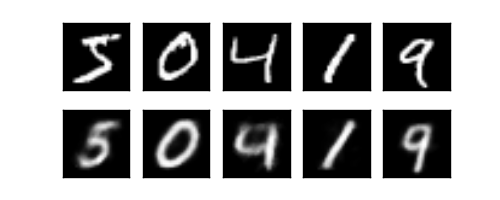所有代码如下：

import torch
import torch.nn as nn
import torch.utils.data as Data
import torchvision
import matplotlib.pyplot as plt
from mpl_toolkits.mplot3d import Axes3D
from matplotlib import cm
import numpy as np

# torch.manual_seed(1)    # reproducible

# Hyper Parameters
EPOCH = 10
BATCH_SIZE = 64
LR = 0.005         # learning rate
N_TEST_IMG = 5

# Mnist digits dataset
train_data = torchvision.datasets.MNIST(
root='./mnist/',
train=True,                                     # this is training data
transform=torchvision.transforms.ToTensor(),    # Converts a PIL.Image or numpy.ndarray to
# torch.FloatTensor of shape (C x H x W) and normalize in the range [0.0, 1.0]
)

# plot one example
print(train_data.train_data.size())     # (60000, 28, 28)
print(train_data.train_labels.size())   # (60000)
plt.imshow(train_data.train_data.numpy(), cmap='gray')
plt.title('%i' % train_data.train_labels)
plt.show()

# Data Loader for easy mini-batch return in training, the image batch shape will be (50, 1, 28, 28)

class AutoEncoder(nn.Module):
def __init__(self):
super(AutoEncoder, self).__init__()

self.encoder = nn.Sequential(
nn.Linear(28*28, 128),
nn.Tanh(),
nn.Linear(128, 64),
nn.Tanh(),
nn.Linear(64, 12),
nn.Tanh(),
nn.Linear(12, 3),   # compress to 3 features which can be visualized in plt
)
self.decoder = nn.Sequential(
nn.Linear(3, 12),
nn.Tanh(),
nn.Linear(12, 64),
nn.Tanh(),
nn.Linear(64, 128),
nn.Tanh(),
nn.Linear(128, 28*28),
nn.Sigmoid(),       # compress to a range (0, 1)
)

def forward(self, x):
encoded = self.encoder(x)
decoded = self.decoder(encoded)
return encoded, decoded

autoencoder = AutoEncoder()

loss_func = nn.MSELoss()

# initialize figure
f, a = plt.subplots(2, N_TEST_IMG, figsize=(5, 2))
plt.ion()   # continuously plot

# original data (first row) for viewing
view_data = train_data.train_data[:N_TEST_IMG].view(-1, 28*28).type(torch.FloatTensor)/255.
for i in range(N_TEST_IMG):
a[i].imshow(np.reshape(view_data.data.numpy()[i], (28, 28)), cmap='gray'); a[i].set_xticks(()); a[i].set_yticks(())

for epoch in range(EPOCH):
for step, (x, b_label) in enumerate(train_loader):
b_x = x.view(-1, 28*28)   # batch x, shape (batch, 28*28)
b_y = x.view(-1, 28*28)   # batch y, shape (batch, 28*28)

encoded, decoded = autoencoder(b_x)

loss = loss_func(decoded, b_y)      # mean square error

if step % 100 == 0:
print('Epoch: ', epoch, '| train loss: %.4f' % loss.data.numpy())

# plotting decoded image (second row)
_, decoded_data = autoencoder(view_data)
for i in range(N_TEST_IMG):
a[i].clear()
a[i].imshow(np.reshape(decoded_data.data.numpy()[i], (28, 28)), cmap='gray')
a[i].set_xticks(()); a[i].set_yticks(())
plt.draw(); plt.pause(0.05)

plt.ioff()
plt.show()

# visualize in 3D plot
view_data = train_data.train_data[:200].view(-1, 28*28).type(torch.FloatTensor)/255.
encoded_data, _ = autoencoder(view_data)
fig = plt.figure(2); ax = Axes3D(fig)
X, Y, Z = encoded_data.data[:, 0].numpy(), encoded_data.data[:, 1].numpy(), encoded_data.data[:, 2].numpy()
values = train_data.train_labels[:200].numpy()
for x, y, z, s in zip(X, Y, Z, values):
c = cm.rainbow(int(255*s/9)); ax.text(x, y, z, s, backgroundcolor=c)
ax.set_xlim(X.min(), X.max()); ax.set_ylim(Y.min(), Y.max()); ax.set_zlim(Z.min(), Z.max())
plt.show()


### 4，变分自动编码器（Variational  Auoencoder）

变分编码器是自动编码器的升级版本，其结构根自动编码器是类似的，也由编码器和解码器构成。

自动编码器就是需要输入一张图片，然后将一张图片编码之后得到一个隐含向量，这比我们随机取一个随机噪声更好，因为这包含着原图片的信息，然后我们隐含向量解码得到与原图片对应的照片。

但是这样我们其实并不能任意生成图片，因为我们没有办法自己去构造隐藏向量，我们需要通过一张图片输入编码我们才知道得到的隐含向量是什么，这时我们就可以通过变分自动编码器来解决这个问题。

其实原理特别简单，只需要在编码过程中给它增加一些限制，迫使其生产的隐含向量能够粗略的遵循一个标准正态分布，这就是与一般的自动编码器最大的不同。

这样我们生产一张新的图片就很简单了，我们只需要给它一个标准正态分布的随机隐含向量，这样通过解码器就能生成我们想要的图片，而不需要给他一张原始图片先编码。

在实际情况中，我们需要在模型的准确率上与隐含向量服从标准正态分布之间做一个权衡，所谓模型的准确率就是指解码器生成的图片与原图片的相似程度。我们可以让网络自己来做这个决定，非常简单，我们只需要将这两者都做一个loss，然后在将他们求和作为总的loss，这样网络就能够自己选择如何才能够使得这个总的loss下降。另外我们要衡量两种分布的相似程度，如何看过之前一片GAN的数学推导，你就知道会有一个东西叫KL divergence来衡量两种分布的相似程度，这里我们就是用KL divergence来表示隐含向量与标准正态分布之间差异的loss，另外一个loss仍然使用生成图片与原图片的均方误差来表示。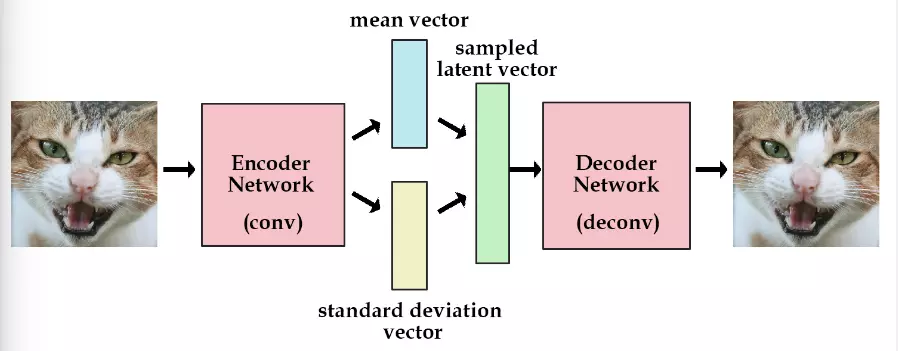下面是PyTorch的实现：
reconstruction_function = nn.BCELoss(size_average=False)  # mse loss

def loss_function(recon_x, x, mu, logvar):
"""
recon_x: generating images
x: origin images
mu: latent mean
logvar: latent log variance
"""
BCE = reconstruction_function(recon_x, x)
# loss = 0.5 * sum(1 + log(sigma^2) - mu^2 - sigma^2)
KLD = torch.sum(KLD_element).mul_(-0.5)
# KL divergence
return BCE + KLD


另外变分编码器除了可以让我们随机生成隐含变量，还能够提高网络的泛化能力、

最后是VAE的代码实现：

class VAE(nn.Module):
def __init__(self):
super(VAE, self).__init__()

self.fc1 = nn.Linear(784, 400)
self.fc21 = nn.Linear(400, 20)
self.fc22 = nn.Linear(400, 20)
self.fc3 = nn.Linear(20, 400)
self.fc4 = nn.Linear(400, 784)

def encode(self, x):
h1 = F.relu(self.fc1(x))
return self.fc21(h1), self.fc22(h1)

def reparametrize(self, mu, logvar):
std = logvar.mul(0.5).exp_()
if torch.cuda.is_available():
eps = torch.cuda.FloatTensor(std.size()).normal_()
else:
eps = torch.FloatTensor(std.size()).normal_()
eps = Variable(eps)

def decode(self, z):
h3 = F.relu(self.fc3(z))
return F.sigmoid(self.fc4(h3))

def forward(self, x):
mu, logvar = self.encode(x)
z = self.reparametrize(mu, logvar)
return self.decode(z), mu, logvar


VAE 的结果比普通的自动编码器要好很多，下面是结果（左边是原图 ，右边是自动编码）：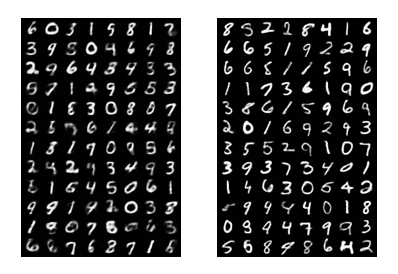VAE的缺点也很明显，他是直接计算生成图片和原始图片的均方误差而不是像GAN那样去对抗来学习，这就使得生成的图片会有点模糊。现在已经有一些工作是将VAE和GAN结合起来，使用VAE的结构，但是使用对抗网络来进行训练，具体可以参考一下这篇论文

### 5，多层感知机学习

在之前学习的 TensorFlow学习笔记——使用TensorFlow操作MNIST数据（1）。我们实现了一个简单的 Softmax Regression 模型，这个线性模型最大的特点是简单易用，但是拟合能力不强。Softmax Regression 可以算是多分类问题 logistic regression ，它进而传统意义上的神经网络的最大区别是哪些没有隐含层。隐含层是神经网络一个重要概念。它是指除输出，输出层外，中间的哪些层。输入层和输出层是对外可见的，因此也可以被称作可视层，而中间层不直接暴露出来，是模型的黑箱部分，通常也比较难具有可解释性，所以一般被称作隐含层。有了隐含层，神经网络就具有了一些特殊的属性，比如引入非线性的隐含层后，理论上只要隐含层节点足够多，即使只有一个隐含层的神经网络也可以拟合任意函数。同时隐含层越多，越容易拟合复杂函数。有理论研究表明，为了拟合复杂函数需要的隐含节点的数目，基本上随着隐含层的数量增多呈指数下降趋势。也就是说层数越多，神经网络所需要的隐含节点可以越少。这也是深度学习的特点之一，层数越深，概念越抽象，需要背诵的知识点（神经网络隐含节点）就越少。不过实际使用中，使用层数较深的神经网络会遇到许多困难，比如容易过拟合，参数难以调试，梯度弥散等等。对这些问题我们需要很多 Trick 来解决，在最近几年的研究中心，越来越多的方法，比如Dropout，Adagrad，ReLUdeng ，逐渐帮助我们解决了一部分问题。

过拟合是机器学习中一个常见的问题，它是指模型预测准确率在训练集上升高，但是在测试集上反而下降了，这通常意味着泛化性不好，模型只是记忆了当前数据的特征，不具备推广能力，尤其是在神经网络中，因为参数众多，经常出现参数比数据还要多的情况，这就非常容易出现只是记忆了训练集特征的情况。为了解决这个问题，Hinton教授团队提出了一个思路简单但是非常有效的方法，Dropout。在使用复杂的卷积神经网络训练图像数据时尤其有效，它的大致思路是在训练时，将神经网络某一层的输出节点数据随机丢弃一部分。我们可以理解为随机把一张图片50%的点删除掉（即随机将50%的点变成黑点），此时人还是很可能识别出这种图片的类型，当时机器也是可以的。这种做法实质上等于创造出了许多新的随机样本，通过增大样本量，减少特征数量来防止过拟合。Dropout 其实也算一种 bagging 方法，我们可以理解成每次丢弃节点数据是对特征的一种采样。相当于我们训练一个 ensemble 的神经网络模型，对每个样本都做特征采样，只不过没有训练多个神经网络模型，只要一个融合的神经网络。

梯度弥散（Gradient Vanishment）是另一个影响深层神经网络训练的问题，在ReLU激活函数出现之前，神经网络训练全部都是用 Sigmoid 作为激活函数。这可能是因为 Sigmoid 函数具有局限性，输出数值在0~1 ，最符合概率输出的定义。非线性的Sigmoid 函数在信号的特征空间映射上，对中央区的信息增益较大，对两侧区的信号增益小。从生物神经科学的角度来看，中央区酷似神经元的兴奋态，两侧区酷似神经元的抑制态。因而在神经网络训练时，可以将重要特征置于中央区，将非中央特征置于两侧区。可以说，Sigmoid比最初期的线性激活函数 y=x ，阶梯激活函数 y=x ，阶梯激活函数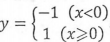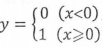好了不少。但是当神经网络层数较多时，Sigmoid函数在反向传播中梯度值会逐渐减少，经过多层的传递后会呈现指数级急剧下降，因此梯度值在传递到前面几层时就变得非常小了。这种情况下，根据训练数据的反馈来更新神经网络的参数将会非常缓慢，基本起不到训练的作用。直到ReLU 的出现，才比较完美的解决了梯度弥散的问题。ReLU是非常简单的非线性函数 Y=max(0, x)，它在坐标轴上式一条折线，当x<=0时，y=0；当x>0时， y=x，非常类似于人脑阈值响应机制。信号在超过某个阈值时，神经元才会进入兴奋和激活的状态，平时则处于抑制状态。ReLU可以很好地传递梯度，经过多层的反向传播，梯度依旧不会大幅缩小，因此非常适合训练很深的神经网络。ReLU从正面解决了梯度弥散的问题，而不需要通过无监督的逐层训练初始化权重来绕行。

ReLU对比 Sigmoid 的主要变化有如下三点：

• 1，单侧抑制
• 2，相对宽阔的兴奋边界
• 3，稀疏激活性

神经科学家在进行大脑能量消耗的研究中发现，神经元编码的工作方式具有稀疏性，推测大脑同时被激活的神经元只有1%~4%。神经元只会对输入信号有少部分的选择性响应，大量不相关的信号被屏蔽，这样可以更高效的提取重要特征。传统的Sigmoid函数则有接近一半的神经元被激活，不符合神经科学的研究。Softplus虽然有单侧抑制，却没有稀疏激活性，因而ReLU函数 max(0, x) 成了最符合实际神经元的模型。目前，ReLU及其变种（EIU34，PReLU35,RReLU36）已经成为了最主流的激活函数。实践中大部分情况下（包含MLP和CNN，RNN内部主要还是使用 Sigmoid，Tanh，Hard Sigmoid）将隐含层的激活函数从 Sigmoid 替换为ReLU都可以带来训练速度及模型准确率的提升。当然神经网络的输出层一般都还是Sigmoid函数，因为他最接近概率输出分布。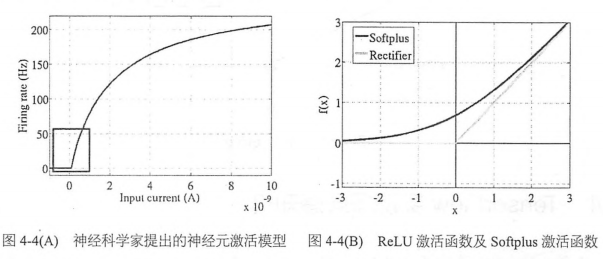那么多层感知器到底是什么呢？下面就介绍一下其原理。

多层感知器（MLP， Multilayer  Perceptron）也叫人工神经网络（ANN，Artificial Neural Network），除了输入输出层，其中间可以有多个隐层，最简单的MLP只含一个隐层，即三层的结构，如下图：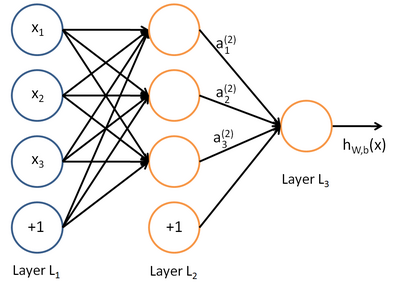从上图可以看到，多层感知机层与层之间是全连接的。多层感知机最底层是输入层，中间是隐藏层，最后是输出层。隐藏层的神经元是怎么得来的？首先他与输入层是全连接的，假设输入层用向量X表示，则隐藏层的输出就是 f(w1x + b1)，w1 是权重（也叫连接系数），b1是偏置，函数f可以是常用的Sigmoid函数或者 tanch函数。

注意1：为什么要使用激活函数

• 1，不使用激活函数，每一层输出都是上层输入的线性函数，无论神经网络有多少层，输出都是输入的线性组合
• 2，使用激活函数，能够给神经元引入非线性因素，使得神经网络可以任意逼近任何非线性函数，这样神经网络就可以利用到更多的非线性模型中

注意2：激活函数需要具备什么性质？

• 1，连续并可导（允许少数点不可导）的非线性函数。可导的激活函数可以直接利用数值优化的方法来学习网络参数
• 2，激活函数机器导数要尽可能的简单，有利于提高网络计算效率
• 3，激活函数的导函数的值域要在一个合适的区间内，不能太大也不能太小，否则会影响训练的效率和稳定性

因此，MLP所有的参数就是各个层之间的连接权重以及偏置，包括 w1, b1, w2, b2.对于一个具体的问题，怎么确定这些参数？求解最佳的参数是一个最优化问题，解决最优化问题，最简单的就是梯度下降法（SGD），即首先随机初始化所有参数，然后迭代地训练，不断地计算梯度和更新参数，直到满足某个条件为止（比如误差足够小，迭代次数足够多时）。这个过程设计到代价函数，规则化（Regularization），学习速率（learning rate），梯度计算等。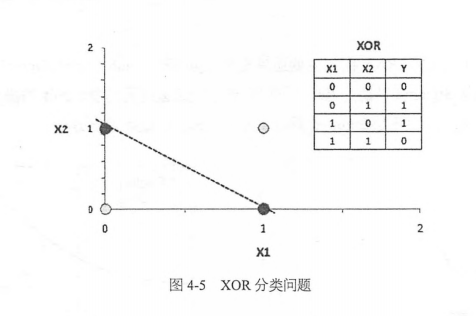### 6，TensorFlow实现多层感知机

代码：

#_*_coding:utf-8_*_
from tensorflow.examples.tutorials.mnist import input_data
import tensorflow as tf

# 创建一个默认的InteractiveSession，这样后面执行各项操作就无须指定Session
sess = tf.InteractiveSession()

in_uints = 784  # 输入节点数
# 在此模型中隐含层的节点数设置在200~1000范围内结果区别都不大
h1_uints = 300  # 隐含层的输出节点数设为300
# W1 b1是隐含层的权重和偏置,将偏置全部赋值为0
# 并将权重初始化为截断的正态分布，其标注差为0.1
W1 = tf.Variable(tf.truncated_normal([in_uints, h1_uints], stddev=0.1))
b1 = tf.Variable(tf.zeros([h1_uints]))
W2 = tf.Variable(tf.zeros([h1_uints, 10]))
b2 = tf.Variable(tf.zeros())

# 由于在训练和预测时，Dropout的比率keep_prob（即保留节点的概率）是不一样的
x = tf.placeholder(tf.float32, [None, in_uints])
keep_prob = tf.placeholder(tf.float32)

# 定义模型结构,神经网络forward时的计算
hidden1 = tf.nn.relu(tf.matmul(x, W1) + b1)
hidden1_drop = tf.nn.dropout(hidden1, keep_prob)
y = tf.nn.softmax(tf.matmul(hidden1_drop, W2) + b2)
y_ = tf.placeholder(tf.float32, [None, 10])
cross_entropy = tf.reduce_mean(-tf.reduce_sum(y_ * tf.log(y),
reduction_indices=))

# 训练模型
tf.global_variables_initializer().run()
for i in range(3000):
batch_xs, batch_ys = mnist.train.next_batch(100)
# 我们加入了keep_prob作为计算图的输入，并在训练时候设为0.75，其余的0.25置为0
train_step.run({x: batch_xs, y_: batch_ys, keep_prob: 0.75})

# 对模型进行准确率评测, 加入一个keep_prob作为输入
# 因为预测部分，所以我们直接令keep_prob=1即可，这样可以达到模型最好的预测效果
correct_prediction = tf.equal(tf.argmax(y, 1), tf.argmax(y_, 1))
accuracy = tf.reduce_mean(tf.cast(correct_prediction, tf.float32))
print(accuracy.eval({x: mnist.test.images, y_: mnist.test.labels,
keep_prob: 1.0}))


训练的时候，这里加入了keep_prob作为计算图的输入，并且在训练时设为0.75，即保留75%的节点，其余的25%置为0。一般来说，对越复杂越大规模的神经网络，Dropout的效果越显著。另外，因为加入了隐含层，我们需要更多的训练迭代来优化模型参数以达到一个比较好的效果。所以一共采用了3000个 batch，每个 batch包含100个样本，一共30万的样本，相当于对全数据集进行了5轮（epoch）迭代。如果增加循环次数，准确率也会略有提高。

结果如下：

0.9778